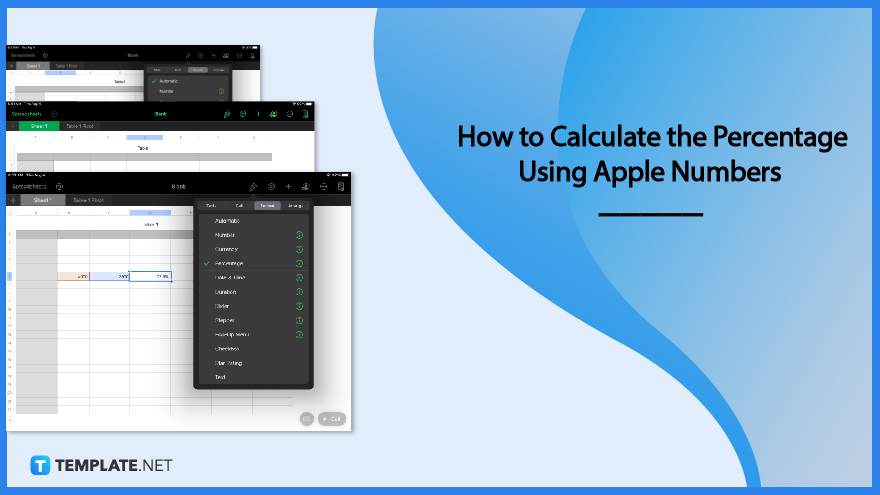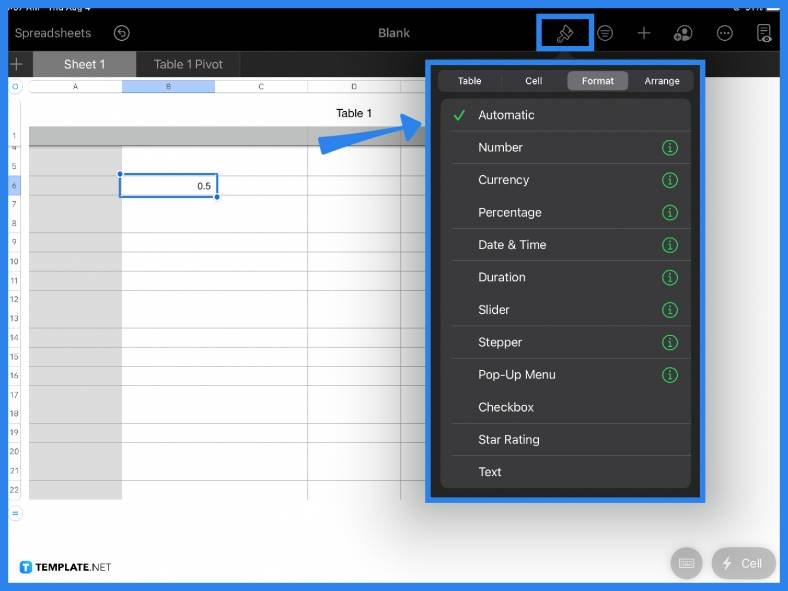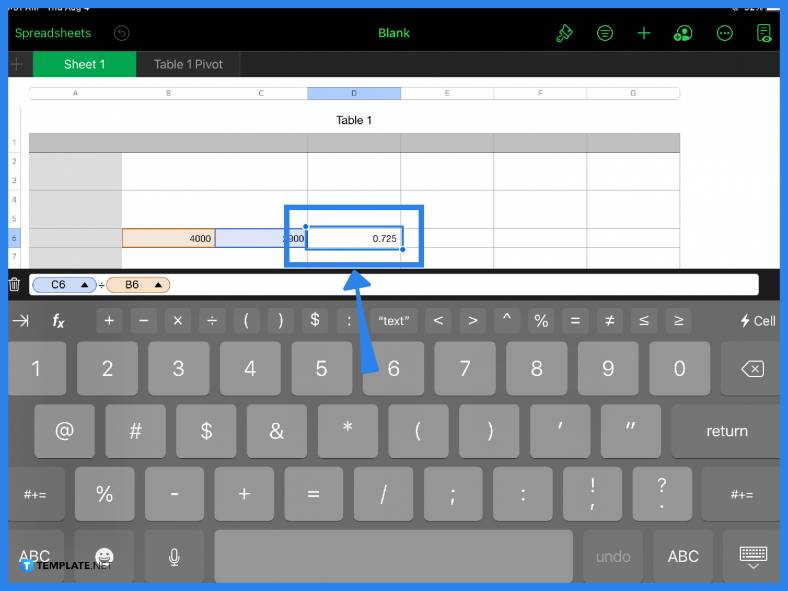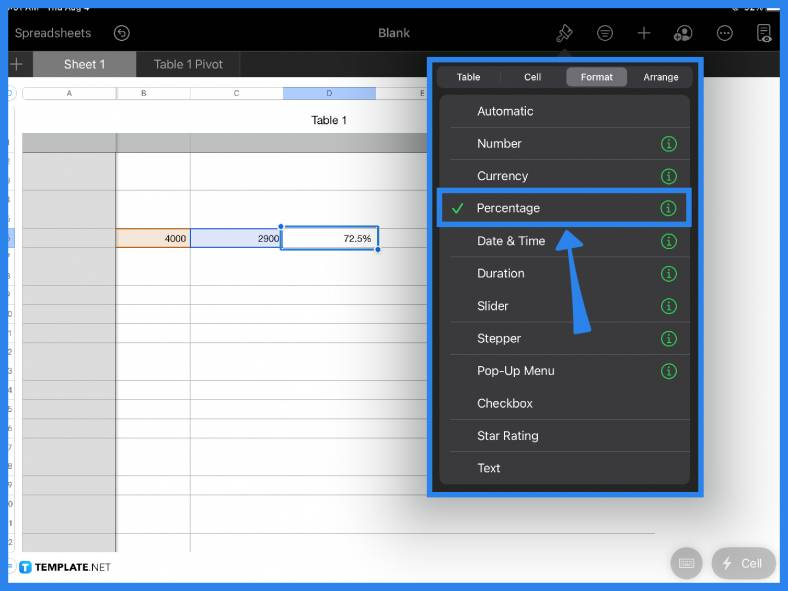# How to Calculate the Percentage Using Apple Numbers

Apple Numbers makes it easy for users to perform mathematical calculations automatically. Getting the percentage of a number or value is essential for business transactions, financial reports, balance sheets, etc.## How to Calculate the Percentage Using Apple Numbers

You do not need to manually calculate the percentage if you are using Apple Numbers. Follow the step-by-step guide below and get the percentage of any value in no time.

• ### Step 1: Use the Formatting ToolIf you need to find the percentage of a single value, you can instantly change a number to percentage format by using the formatting tool. Apple Numbers comes with a range of different formats in its drop-down menu. For example, if you type in a decimal amount in a cell, simply click on it, select the paintbrush tool on the main toolbar, then choose Percentage.

• ### Step 2: Type the Equal SignIf you need to find the percentage of two or more values then you need to utilize the formula bar. Take note of the values you want to calculate and their cell locations. Click on your destination cell and type in the equal (=) sign. This automatically opens up the formula bar. Key in the cell name of your first value, add in the necessary mathematical function, and then type in the second value. If you have a longer formula or you have more cells to include, the same process applies.

• ### Step 3: Hit Enter or Click the Green Check MarkWhen you have factored in your formula and values, hit Enter or the green check mark in the formula bar to get the answer. On your spreadsheet, if the result is not in percentage form, simply click on it and select the paintbrush tool then under the Format menu, select Percentage.

• ### Step 4: Use the Formula Bar

This last step is another alternative to get the percentage. Aside from doing the steps above, you can also get the percentage of a cell by typing the = sign to open the formula bar, inputting the cell name and selecting the % function found in the formula bar.

## FAQs

### How do you do percentages on Apple Numbers?

The fastest and most convenient way to do percentages on Apple Numbers is by utilizing the formula bar; simply key in your values and use the % tool in the formula bar.

### How do you write a percentage on a Mac?

On macOS, you can insert the % sign found on the keyboard or, alternatively, you can use the keyboard shortcut which is Option + 0025.

### How do you add a formula in Apple Numbers?

To add a formula in Apple Numbers, click a cell and type in the equal (=) sign, insert the values, and your chosen arithmetic operator (e.g., addition, subtraction, etc.)

### What is a percentage and its formula?

A percentage is a number or value that is expressed in terms of 100 or as a fraction of a hundred.

### How do you calculate percentages quickly?

To calculate quickly, the most practical and convenient way is either to use a calculator or the built-in percentage function in spreadsheet software applications such as Apple Numbers.